# Anharmonic Oscillator

## Recommended Posts

Instead of trying to force Physics to conform to your own ideas how about we try something different, because you will not succeed.

You want an oscillator.

Can you list the what happens to the velocity, acceleration and position of any oscillator as it runs through one complete cycle?

• Replies 276
• Created

#### Popular Posts

Sigh no you have to recognize that harmonic, inharmonic and anharmonic are descriptives of the characteristics of the oscillator.  Here is how classical harmonic oscillator is described.  A

The gauge groups I mentioned are not specifically the harmonic oscillator. QFT accounts for the quantum harmonic oscillator in its gauge groups but that isn't the full story.  https://www.google.

That’s the equation, but it’s not the solution, which would give you x(t)

#### Posted Images

3 minutes ago, studiot said:

Can you list the what happens to the velocity, acceleration and position of any oscillator as it runs through one complete cycle?

I did not understand the question. It is a particle that makes a round trip with the constraint of the contraction / relaxation of the spring.

##### Share on other sites
14 minutes ago, Kartazion said:

I did not understand the question. It is a particle that makes a round trip with the constraint of the contraction / relaxation of the spring.

So to use velocity as an example.

Is the velocity the same (ie constant) throughout the cycle?

Is the velocity ever zero ?

Is there a maximum velocity?

Is the velocity continuous ie are there any jumps or sudden changes ?

If the answers to any of these questions is yes then at what point in the cycle does this happen?

and so on.

Similar questions can be applied to acceleration and position or displacement.

##### Share on other sites
2 minutes ago, studiot said:

If the answers to any of these questions is yes then at what point in the cycle does this happen?

There is a maximum Velocity at x = 0.

I understood. We can extend the y axis as much as we want. Which brings us to a sharp acceleration.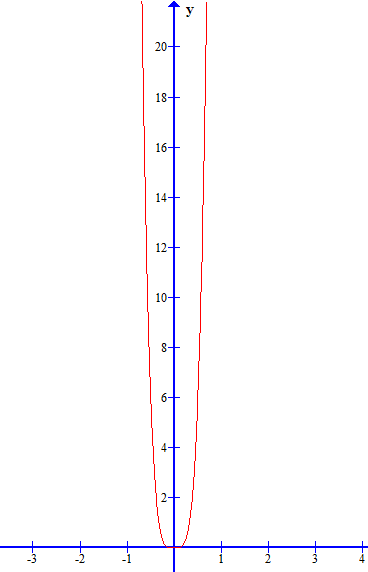##### Share on other sites
34 minutes ago, Kartazion said:

There is a maximum Velocity at x = 0.

I understood. We can extend the y axis as much as we want. Which brings us to a sharp acceleration.

So what about the other questions?

You need to know the answers to all of them and more to fully understand how any (repeat any) oscillator works.

This is why is is common to start by studying the simple harmonic oscillator, which is very easy.

I use MathCad, Excel, Geogebra and others.

But the plots came from wolframalpha, which is a free maths site and very useful.

Just type plot y = ....... into the input box and sit back.

##### Share on other sites
1 hour ago, studiot said:

So what about the other questions?

You need to know the answers to all of them and more to fully understand how any (repeat any) oscillator works.

This is why is is common to start by studying the simple harmonic oscillator, which is very easy.

Yes thank you. In any case your help was precious to me.

I thought that I can repeat the cycle with dx / dt where (t) follow x with a kind of table. The value of t gives the number of cycles.

My question is: can I to write the initial conditions like that: 1 second = 1 complete cycle of x ?

1 hour ago, studiot said:

I use MathCad, Excel, Geogebra and others.

But the plots came from wolframalpha, which is a free maths site and very useful.

Just type plot y = ....... into the input box and sit back.

It's awesome this link, thanks again.

Edited by Kartazion
##### Share on other sites

After several attempts I concluded that the value of b defines the size of the oscillator according to the total magnitude of x (several meters or a few millimeters), without losing the ratio of the proportionality between distance and speed and acceleration.I want to make big and / or small oscillator, I'm talking about the size of the length of x, the distance that separates the two ends of the particle.
So I wish to change the equation, and rewrite it as follows:

With a virtual particle and a virtual force, and if d is smaller or equal than to 0.01, can we define an oscillator like this?

$$\frac{d^2 x}{dt^2}+\sqrt{x^2-bx^4}=0$$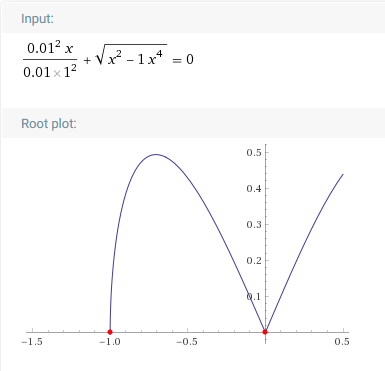If yes, can we compute v in the same way with:

$$2\frac{{dx}}{{dt}}\frac{{{d^2}x}}{{d{t^2}}} = ...$$

Originally it was to find v we raised AKx³ to bx^4.

Edited by Kartazion
LaTeX
##### Share on other sites

15 hours ago, Kartazion said:

My question is: can I to write the initial conditions like that: 1 second = 1 complete cycle of x ?

You are ignoring Dr Swanson's reply from a much earlier post when you asked this question before.

On 11/22/2019 at 11:20 AM, swansont said:

Note that you can't arbitrarily change the frequency of the oscillation.

However I'm glad to se you finally have your plots the right way up to talk about oscillators.

Do you know what equilibrium means and how it is important to any oscillator?

Edited by studiot
##### Share on other sites
16 hours ago, studiot said:

Do you know what equilibrium means and how it is important to any oscillator?

I do not know exactly. I think it's still at x = 0 that we have an equilibrium position.

Otherwise I have this if x = 0 ,  v = 0 ,  a = 1 ,  b = 1I know that I use a virtual oscillator that does not take into account Newton's laws with my plot.

Afterwards, the race of the particle in the potential well is precisely related to the maximum energy.
Now I know and in the case of a harmonic oscillator, and with the Newton's laws, that the potential energy is related to:

$Ep(x)=\frac{1}{2}Kx^2$

And in the case of an anharmonic oscillator:

$Ep(x)=\frac{1}{2}Kx^2 - \frac{1}{3}AKx^3$

But at the extremity of x (at i and j) there is also an equilibrium position that is created? No ? Where the particle slowed down to the max for after going back in the other direction.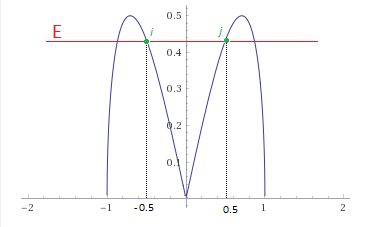Edited by Kartazion
##### Share on other sites
54 minutes ago, studiot said:

Sadly since you are not listening to the bulk of what is said to you, there is no point saying any more.

I confess that I do not understand what error of advice I have not followed.

##### Share on other sites

#######################

I saw on a thread a link, here, which shows this potential energy between a1 and a2.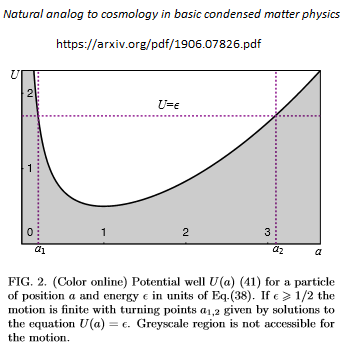From arXiv here, I read "The Coulomb system of identical particles obeys equations that have the structure of the cosmological equations of the general theory of relativity."

This is the part of the general theory of relativity that made me think, because my question is:
Can we rally the Coulomb-Newton correspondence relation with the anharmonic oscillator?
If yes, is there a direct relationship with the potential energy from $$Ep(x)=\frac{1}{2}Kx^2$$ ?

##### Share on other sites

None of the mathematics in that paper describes an anharmonic oscillator. I read the paper as it was posted in another thread and I wouldn't place any faith in it. It relies on a negative cosmological constant which there is zero evidence for such.

##### Share on other sites
17 minutes ago, Mordred said:

None of the mathematics in that paper describes an anharmonic oscillator. I read the paper as it was posted in another thread and I wouldn't place any faith in it. It relies on a negative cosmological constant which there is zero evidence for such.

Ok.

Otherwise I found that: Connection between Coulomb and harmonic oscillator potentials in relativistic quantum mechanics

Edited by Kartazion
##### Share on other sites

That's a better quality paper, the harmonic oscillator is fundamental in QM. So you can find plenty of information on it.

##### Share on other sites
On 12/16/2019 at 7:51 AM, Mordred said:

... the harmonic oscillator is fundamental in QM. So you can find plenty of information on it.

In what other area of quantum mechanics are we using precisely the harmonic oscillator?

1 - In solids with the vibration of atoms, where if we move the atoms slightly away from its equilibrium position, a restoring force will try to put the molecule back in the equilibrium position.

2 - For coherent states, among others used to describe the states of light of lasers.

and what other domain?

Thank you

##### Share on other sites

Multi particle systems Incorporated with the Heisenberg uncertainty principle. When I get off work I will post the relevant formulas.

##### Share on other sites

I have just found here some good equations concerning the uncertainty principle. Which brings me to two questions.

The first concerns the equation of the minimum energy of the oscillator. In normal times we cannot simultaneously to know the position and the momentum of the subatomic particles.
The question is: the uncertainty principle also applies on the quantum harmonic oscillator with \Delta x and \Delta p ? Is this what makes the difference with the classical harmonic oscillator?

My second question is: why do we use the Planck constant so often in the equations of the quantum harmonic oscillator to express the principle of uncertainty?

Edited by Kartazion
LaTeX
##### Share on other sites

Ok you found the relevant formulas for the quantum harmonic oscillator so I won't need to post them.

The Planck constant is applied due to the quantization wave nature of all particles. All particles have discreet wave functions this means only that wavelengths divisible by the Planck constant are possible.

The zero point energy essentially means that absolute zero is not viable. There is always harmonic oscillations and thus a minimal non zero energy state present.

In classical harmonic oscilators there is no uncertainty in position or momentum the uncertainty applies in the quantum but not classical regime.

##### Share on other sites
5 hours ago, Mordred said:

Ok you found the relevant formulas for the quantum harmonic oscillator so I won't need to post them.

It is thanks to your help.

5 hours ago, Mordred said:

The Planck constant is applied due to the quantization wave nature of all particles. All particles have discreet wave functions this means only that wavelengths divisible by the Planck constant are possible.

Ok

5 hours ago, Mordred said:

The zero point energy essentially means that absolute zero is not viable. There is always harmonic oscillations and thus a minimal non zero energy state present.

Yes it's always on the move.

5 hours ago, Mordred said:

In classical harmonic oscilators there is no uncertainty in position or momentum the uncertainty applies in the quantum but not classical regime.

Of course, in classical physics we can always determine the two values simultaneously.

###################

Otherwise I would have a weird calculation to do.

I want to know if it is possible to have an infinitesimal interval ℏ and express the velocity as:

$$\frac{\hslash x}{\hslash t}$$

I don't think so, but I wish to be able to calculate the oscillator in an unusual direction. Example:##### Share on other sites
8 hours ago, Mordred said:

The zero point energy essentially means that absolute zero is not viable.

I always understood that this was the other way around.

That is the zero point energy was introduced because of the uncertainty principle since at the zero point both uncertainty variables, in a pair, would be uniquely determined without it.

20 hours ago, Kartazion said:

In what other area of quantum mechanics are we using precisely the harmonic oscillator?

Used in spectroscopy to account for line broading/emission time/absorbtion time in spectroscopy resolution.

##### Share on other sites
3 hours ago, studiot said:

I always understood that this was the other way around.

That is the zero point energy was introduced because of the uncertainty principle since at the zero point both uncertainty variables, in a pair, would be uniquely determined without it.

The energy of a harmonic oscillator is (n+1/2) hbar*omega — energy is there even in the n=0 state. It's consistent with the HUP, of course, as it must, but the zero point energy drops directly out of the solution. It's not something that was added on because of the HUP (which is how "introduced" makes it sound, to me)

##### Share on other sites
20 minutes ago, swansont said:

The energy of a harmonic oscillator is (n+1/2) hbar*omega — energy is there even in the n=0 state. It's consistent with the HUP, of course, as it must, but the zero point energy drops directly out of the solution. It's not something that was added on because of the HUP (which is how "introduced" makes it sound, to me)

This is how the history of the 'half' was presented to me back in th 1960s.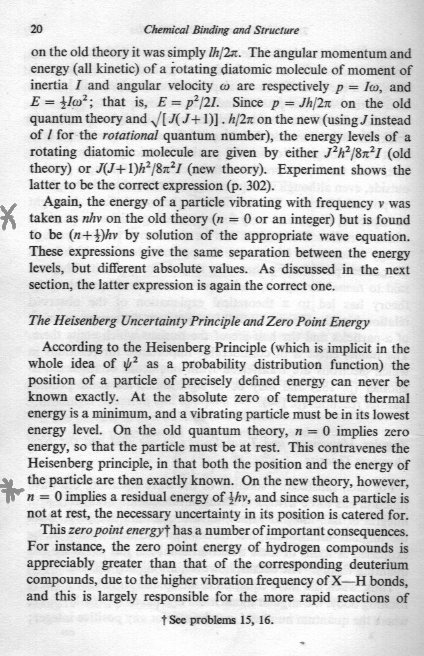Edited by studiot

## Create an account

Register a new account